# Mean-values

There are so many results and conjectures having to do with the mean-values or moments of L-functions in families that it is difficult to mention all of them. So we will stick with some of the more familiar ones.

Moments of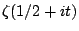. Let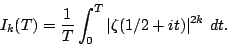Then asymptotic formulae are known for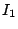and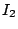. A good estimation for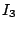seems to be far out of reach of today's technology (maybe we need to know more about automorphic forms on GL3?) but would be a very important milestone. Conjectures based on number theory are known for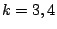. Conjectures based on random matrix theory are known for all real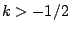.

Moments of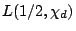. Let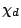be a real, primitive, quadratic character to the modulus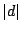(i.e. a Kronecker symbol). Let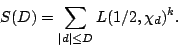Then asymptotics are known for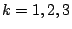. A conjecture based on number theory is known for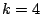. Conjectures based on random matrix theory are known for all real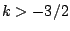. The fourth moment is just slightly out of reach of current technology.

Moments of automorphic L-functions. Let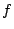be a normalized newform of weight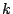and level 1 and let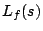be the associated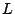-function (with critical strip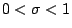.) Let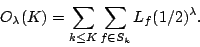Then asymptotic formulae are known for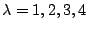. Conjectures based on random matrix theory are known for all real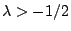. Is the fifth moment doable?

Quadratic twists of automorphic L-functions. Letbe a fixed newform (for example the newform associated with a given elliptic curve). Letbe the associated L-function and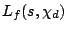the twist by the primitive quadratic character. It is possible to evaluate the first moment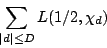but can one do the second moment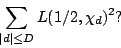Again, this is just at the edge of what can be done by today's techniques and is a problem worthy of study.

Special families. Recently the bound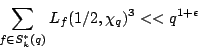has been obtained, where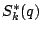denotes the set of newforms of weightand level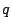(no character), and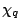is the real character to the moduluswhereis odd and squarefree. This estimate has been used to bound fourier coefficients of half-integral weight forms and (its analogue for Maass forms has been used) to bound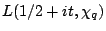. Can one strengthen this estimate to give an asymptotic formula (perhaps forrestricted to prime values)? Are there other situations where the underlying arithmetic is so fortuitous so as to give such a strong bound?

Back to the main index for L-functions and Random Matrix Theory.